# Clipper and Clamper Circuits

## Discrete Semiconductor Devices and Circuits

• #### Question 1

 Don’t just sit there! Build something!!

Learning to mathematically analyze circuits requires much study and practice. Typically, students practice by working through lots of sample problems and checking their answers against those provided by the textbook or the instructor. While this is good, there is a much better way.

You will learn much more by actually building and analyzing real circuits, letting your test equipment provide the “answers” instead of a book or another person. For successful circuit-building exercises, follow these steps:

1. Carefully measure and record all component values prior to circuit construction, choosing resistor values high enough to make damage to any active components unlikely.
2. Draw the schematic diagram for the circuit to be analyzed.
3. Carefully build this circuit on a breadboard or other convenient medium.
4. Check the accuracy of the circuit’s construction, following each wire to each connection point, and verifying these elements one-by-one on the diagram.
5. Mathematically analyze the circuit, solving for all voltage and current values.
6. Carefully measure all voltages and currents, to verify the accuracy of your analysis.
7. If there are any substantial errors (greater than a few percent), carefully check your circuit’s construction against the diagram, then carefully re-calculate the values and re-measure.

When students are first learning about semiconductor devices, and are most likely to damage them by making improper connections in their circuits, I recommend they experiment with large, high-wattage components (1N4001 rectifying diodes, TO-220 or TO-3 case power transistors, etc.), and using dry-cell battery power sources rather than a benchtop power supply. This decreases the likelihood of component damage.

As usual, avoid very high and very low resistor values, to avoid measurement errors caused by meter “loading” (on the high end) and to avoid transistor burnout (on the low end). I recommend resistors between 1 kΩ and 100 kΩ.

One way you can save time and reduce the possibility of error is to begin with a very simple circuit and incrementally add components to increase its complexity after each analysis, rather than building a whole new circuit for each practice problem. Another time-saving technique is to re-use the same components in a variety of different circuit configurations. This way, you won’t have to measure any component’s value more than once.

• #### Question 2

What type of electronic component do these symbols represent, and what special function does it perform?• #### Question 3

Explain how a surge protector functions: the kind of device used to protect electronic equipment against common power line voltage transients. Draw a schematic diagram to accompany your explanation.

• #### Question 4

A technician builds her own audio test set for use in troubleshooting audio electronic circuitry. The test set is essentially a sensitive detector, allowing low-power audio signals to be heard:What purpose do the two diodes serve in this circuit? Hint: if you remove the diodes from the circuit, you will not be able to hear the difference in most cases!

• #### Question 5

Predict how the operation of this sensitive audio detector circuit will be affected as a result of the following faults. Consider each fault independently (i.e. one at a time, no multiple faults):Diode D1 fails open:
Diode D1 fails shorted:
Transformer T1 primary winding fails open:
Resistor R1 fails open:
Solder bridge (short) past resistor R1:
Wiper fails to contact slide in potentiometer:

For each of these conditions, explain why the resulting effects will occur.

• #### Question 6

Determine both the waveshape and amplitude of the AC signal measured by the oscilloscope at the output of this circuit: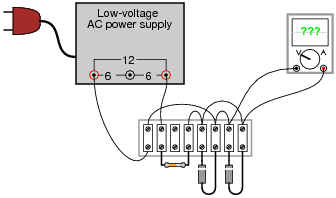The diodes are model 1N4001, each. The resistor’s color code is Brown, Black, Orange, Silver.

• #### Question 7

Sketch the shape of the output voltage waveform for this “clipper” circuit, assuming an ideal diode with no forward voltage drop: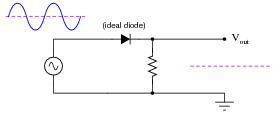• #### Question 8

Sketch the shape of the output voltage waveform for this “clipper” circuit, assuming an ideal diode with no forward voltage drop: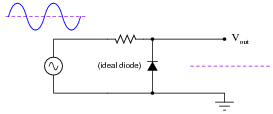• #### Question 9

Sketch the shape of the output voltage waveform for this “clipper” circuit, assuming an ideal diode with no forward voltage drop: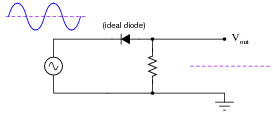• #### Question 10

Sketch the shape of the output voltage waveform for this “clipper” circuit, assuming an ideal diode with no forward voltage drop: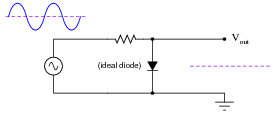• #### Question 11

Predict how the operation of this clipper circuit will be affected as a result of the following faults. Consider each fault independently (i.e. one at a time, no multiple faults):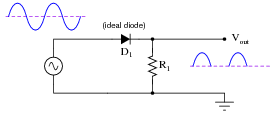Diode D1 fails open:
Diode D1 fails shorted:
Resistor R1 fails open:
Resistor R1 fails shorted:

For each of these conditions, explain why the resulting effects will occur.

• #### Question 12

Predict how the operation of this clipper circuit will be affected as a result of the following faults. Consider each fault independently (i.e. one at a time, no multiple faults):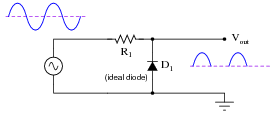Diode D1 fails open:
Diode D1 fails shorted:
Resistor R1 fails open:
Resistor R1 fails shorted:

For each of these conditions, explain why the resulting effects will occur.

• #### Question 13

Design a clipper circuit that eliminates the positive portion of this AC waveform, leaving only the negative half-cycles to appear on the output: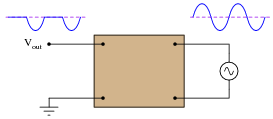• #### Question 14

Design a clipper circuit that clips any portion of the input AC waveform below 4 volts: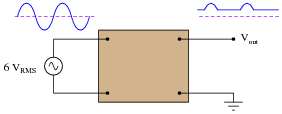• #### Question 15

Describe what happens to the shape of the load voltage waveform when the potentiometer is adjusted in this clipper circuit:• #### Question 16

There is a problem with this clipper circuit, as evidenced by the output waveform: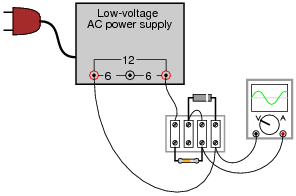What is the most likely cause of this problem, and how would you verify your conclusion with further measurements?

• #### Question 17

The simplest form of AM radio receiver is the so-called crystal receiver circuit. It gets its name from the very early days of solid-state electronics, when crude signal rectifying diodes were constructed from certain types of mineral crystals:Explain how the AM radio signal becomes “demodulated” into an audio-frequency signal, through the clipping action of the diode.

• #### Question 18

Clamper circuits are sometimes referred to as DC restorer circuits. Explain why.

Does a “clamper” circuit change the shape of a voltage waveform, like a “clipper” circuit does? Explain why or why not.

• #### Question 19

Draw the output waveform shape for this circuit, assuming an ideal diode (no forward voltage drop and no reverse leakage):• #### Question 20

Design a clamper circuit that biases the AC waveform so it lies completely below (negative) the zero line: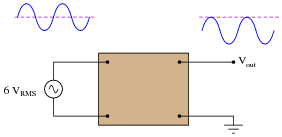• #### Question 21

In this circuit, the values of capacitor C1 and resistor R1 are chosen to provide a short time constant, so they act as a differentiator network. This results in a brief pulse of voltage across R1 at each leading edge of the square wave input. Capacitor C2 and resistor R2 are sized to provide a long time constant, so as to form an integrator network. This time-averages the brief pulses into a final DC output voltage relatively free of ripple.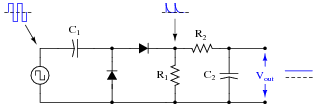Explain what happens to the output voltage as the input frequency is increased, assuming the input voltage amplitude does not change. Can you think of any practical applications for a circuit such as this?